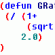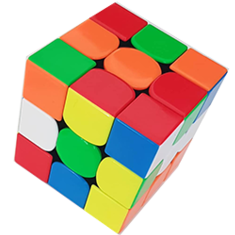# Lisp routine to round off text numbers in autocad (25.362~25.360) .. how to avoid 0 ?## Recommended PostsThis lisp routine is to set decimal digits after a comma in any integer to 2 digit for any set of entries even in term of text ,, how to avoid the appearance of 0 .. ( ex : 25.456 ~25.460 , 25.322~25.320 ) last 0 isn't preferable ..

; Program to add specific value to all the selected texts

(defun c:ROFF()

(setq selset(ssget (list (Cons 0 "TEXT"))))

(setq len(sslength selset))

(setq ctr 0)

(repeat len

(setq ent(Ssname selset ctr))

(setq ctr(1+ ctr))

(setq entl(entget ent))

(setq txtv(Atof (cdr (assoc 1 entl))))

(setq remval(- (fix (* txtv 1000)) (* 10 (fix (* txtv 100)))))

(setq fixedv(fix (* txtv 1000)))

(if (= remval 0) (setq fixn (+ fixedv 0)))

(if (= remval 1) (setq fixn (- fixedv 1)))

(if (= remval 2) (setq fixn (- fixedv 2)))

(if (= remval 3) (setq fixn (- fixedv 3)))

(if (= remval 4) (setq fixn (- fixedv 4)))

(if (= remval 5) (setq fixn (+ fixedv 5)))

(if (= remval 6) (setq fixn (+ fixedv 4)))

(if (= remval 7) (setq fixn (+ fixedv 3)))

(if (= remval(setq fixn (+ fixedv 2)))

(if (= remval 9) (setq fixn (+ fixedv 1)))

(setq fixn(atof (rtos (/ fixn 1000.00) 2 )))

(setq sub(subst (Cons 1 (rtos fixn 2 )) (assoc 1 entl) entl))

(entmod sub)

(entupd ent)

)

(princ)

)

(princ "\nType \"ROFF\" at the command prompt") (princ)

##### Share on other sitesemotionshould be replaced with

8 )
without space.
##### Share on other sitesLook into DIMZIN sysvar -David

##### Share on other sitesHere ya go...an old code i found.

Seemed to work with my single test on Acad 2011.

Just type NP, enter your precision and select the text/mtext.

```;4-9-09
;Implements user specified precision on integers with or without text
;used getPrec by CAB
(defun c:NP (/ RtosPrec ss x Number Prec charlist n snumber numlist char strippednumber nnumber NewString)
(setq RtosPrec (getint "Enter Precision to force on all Text and MText Integers:"))
(setq ss (ssget '((-4 . "<OR")(0 . "TEXT")(0 . "MTEXT")(-4 . "OR>"))))
(setq x 0)
(Repeat (sslength ss)
(progn
(setq Number (ssname ss x))
(defun getPrec (str)
(if (vl-string-search "/" str)
(setq str (vl-string-right-trim "0" (rtos (distof str) 2 ))
)
(if (vl-string-search "." str)
(- (strlen str)(vl-string-search "." str) 1)
0
)
)
(setq Prec (getprec (cdr (assoc 1 (entget Number)))))
(setq charlist (vl-string->list (cdr (assoc 1 (entget Number)))))
(setq n 0 snumber nil)
(setq numlist (list "0" "1" "2" "3" "4" "5" "6" "7" "8" "9"))
(repeat (length charlist)
(setq char (chr (nth n charlist)))
(if (member char numlist)
(setq snumber (append snumber (list char)))
);if
(setq n (1+ n))
);repeat
(if snumber
(progn
(setq StrippedNumber 0)
(setq n (length snumber))
(setq char 0)
(repeat (- n prec)
(setq StrippedNumber (+ StrippedNumber (* (atoi (nth char snumber))(expt 10 (1- (- n prec))))))
(setq n (1- n)
char (1+ char)
);setq
);repeat
(setq n prec)
(setq char (length snumber))
(repeat prec
(setq StrippedNumber (+ StrippedNumber (* (atoi (nth (1- char) snumber))(expt 0.1 n))))
(setq n (1- n)
char (1- char)
);setq
);repeat
(setq snumber (rtos strippednumber 2 prec))
(setq nnumber (rtos strippednumber 2 rtosprec))
(setq NewString
(strcat
(substr (cdr (assoc 1 (entget Number))) 1 (- (length (vl-string->list (cdr (assoc 1 (entget Number)))))(length (vl-string->list snumber))))
nnumber
)
)
(entmod (subst (cons 1 NewString)(assoc 1 (entget Number)) (entget Number)))
);progn
);if
);progn
(setq x (1+ x))
);repeat
);defun
```

##### Share on other sitesemotionshould be replaced with without space.

Salama, please note that in advanced editor (Go advanced button at bottom of post input field, you will find a check box that will allow you to disable the automated smilies recognition in posts.

Also, please consider using the Code tags instead of Quote ones when posting code excerpt; this will ensure keeping the formatting.

Edited by MSasu
Removed some formatting issues
##### Share on other sitesSalama,

You could save a lot of "setq" use by using a "cond" statment see example below;

Modified Code;

```(setq fixn
(cond
((= remval 0)  (+ fixedv 0))
((= remval 1)  (- fixedv 1))
((= remval 2)  (- fixedv 2))
((= remval 3)  (- fixedv 3))
((= remval 4)  (- fixedv 4))
((= remval 5)  (+ fixedv 5))
((= remval 6)  (+ fixedv 4))
((= remval 7)  (+ fixedv 3))
((= remval   (+ fixedv 2))
((= remval 9)  (+ fixedv 1))
)
)
```

```(if (= remval 0) (setq fixn (+ fixedv 0)))
(if (= remval 1) (setq fixn (- fixedv 1)))
(if (= remval 2) (setq fixn (- fixedv 2)))
(if (= remval 3) (setq fixn (- fixedv 3)))
(if (= remval 4) (setq fixn (- fixedv 4)))
(if (= remval 5) (setq fixn (+ fixedv 5)))
(if (= remval 6) (setq fixn (+ fixedv 4)))
(if (= remval 7) (setq fixn (+ fixedv 3)))
(if (= remval 8 (setq fixn (+ fixedv 2)))
(if (= remval 9) (setq fixn (+ fixedv 1)))
```

##### Share on other sitesor

```(setq fixn (eval
(nth	remval
'((+ fixedv 0)
(- fixedv 1)
(- fixedv 2)
(- fixedv 3)
(- fixedv 4)
(+ fixedv 5)
(+ fixedv 4)
(+ fixedv 3)
(+ fixedv 2)
(+ fixedv 1)
)
)
)
)```

or

```(setq fixn (eval (cadr
(assoc remval
'((0 (+ fixedv 0))
(1 (- fixedv 1))
(2 (- fixedv 2))
(3 (- fixedv 3))
(4 (- fixedv 4))
(5 (+ fixedv 5))
(6 (+ fixedv 4))
(7 (+ fixedv 3))
(8 (+ fixedv 2))
(9 (+ fixedv 1))
)
)
)
)
)```

```(if (and
(< -1 fixedv 10)
fixedv) (...))```

##### Share on other sitesOr:

```(if (< remval 5)
(setq fixn (- fixedv remval))
(setq fixn (+ fixedv (- 10 remval)))
)```

##### Share on other sitesOr:

```(if (< remval 5)
(setq fixn (- fixedv remval))
(setq fixn (+ fixedv (- 10 remval)));[b][color="blue"];<-- brilliant[/color][/b]
)```

really?oh man, did you put a lot of thought on that one? or it only took you a minute to come up with that?

##### Share on other sitesreally?oh man, did you put a lot of thought on that one? or it only took you a minute to come up with that?

Thanks pBe - I spent some time trying to avoid the if statement, but the pattern for 5 and above was quite simple to spot as both numbers sum to 10 in each case## Join the conversation

You can post now and register later. If you have an account, sign in now to post with your account.
Note: Your post will require moderator approval before it will be visible.Unfortunately, your content contains terms that we do not allow. Please edit your content to remove the highlighted words below.

×   Pasted as rich text.   Restore formatting

Only 75 emoji are allowed.